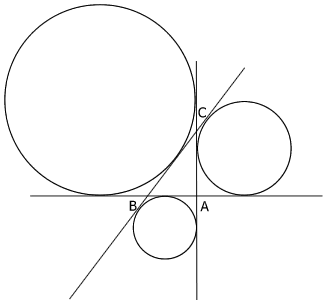#### You may also like### Baby Circle

A small circle fits between two touching circles so that all three circles touch each other and have a common tangent? What is the exact radius of the smallest circle?### Kissing

Two perpendicular lines are tangential to two identical circles that touch. What is the largest circle that can be placed in between the two lines and the two circles and how would you construct it?### Logosquares

Ten squares form regular rings either with adjacent or opposite vertices touching. Calculate the inner and outer radii of the rings that surround the squares.

# Escriptions

##### Age 16 to 18Challenge Level

The idea for this problem was suggested by Geoff Faux. See also the NRICH Problem 'Polycircles' .

 Try to visualise necklaces of touching circles centred at the vertices of a right-angled triangle, first where the circles touch on the sides of the triangle and then where points of contact lie on the sides produced. Now try to visualise where these circles meet the inscribed and escribed circles of the triangle. Given any right-angled triangle $ABC$ with sides $a, b$ and $c$, find the radii of the three circles with centres at $A, B$ and $C$ such that each circle touches the other two and two of the circles touch on $AB$ between $A$ and $B$, two circles touch on $CA$ produced and two circles touch on $CB$ produced. Now for any right-angled triangle $ABC$ find the radii of the three escribed circles as shown in the diagram.